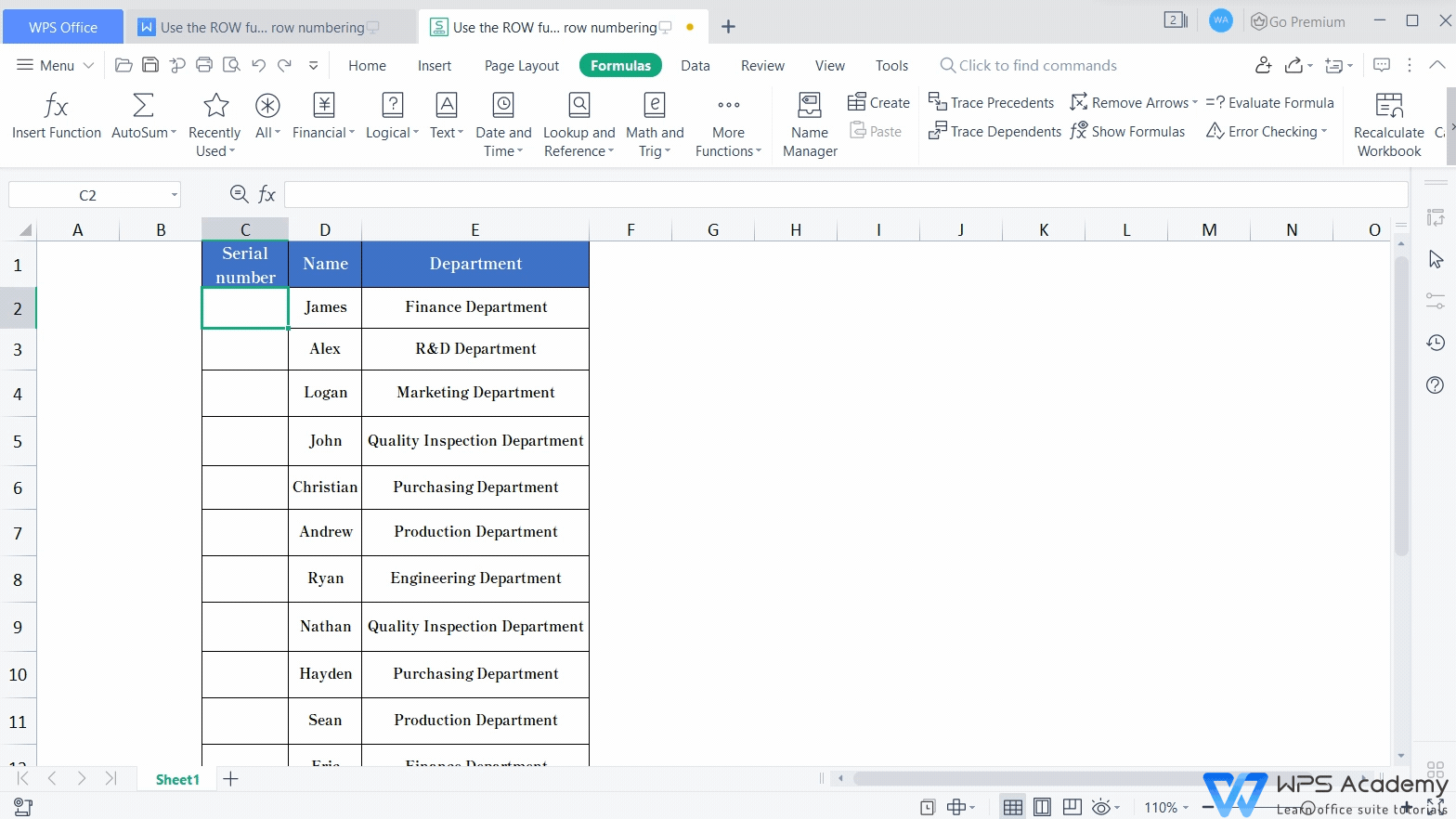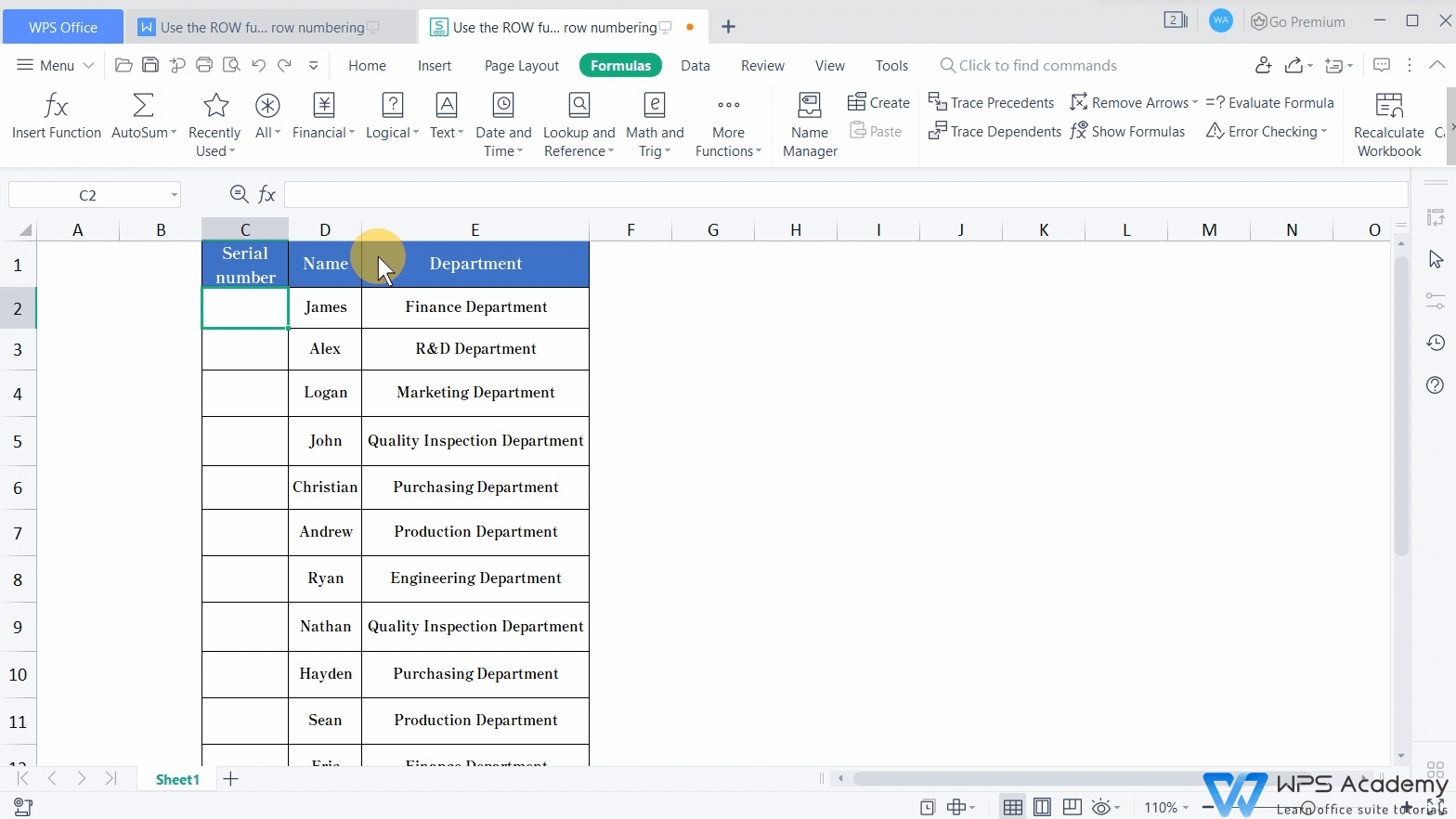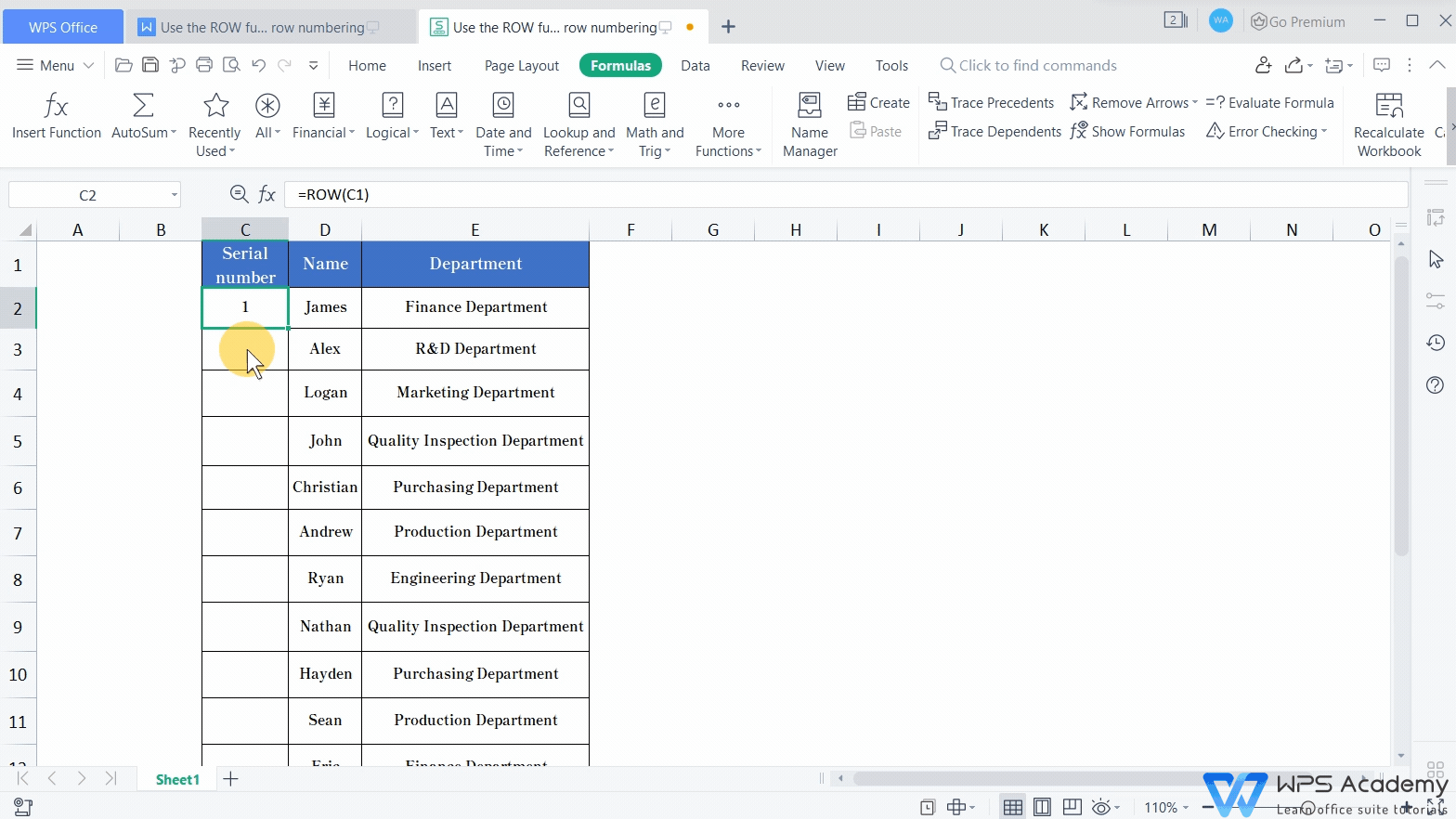Use the ROW function in WPS Spreadsheets for row numbering

Uploaded time: August 27, 2021 Difficulty: PrimaryUse the ROW function in WPS Spreadsheets for row numbering

Tips: you can turn on the volume by clicking

Graphic skills

When using WPS Spreadsheets to process data, how can we deal with the worksheets without numbering or those with disordered numbers? Are there any convenient ways to number the rows while the numbers are not supposed to get disordered when certain rows are removed? The ROW function can realize it.

The complete ROW formula is =ROW([Reference]). Here Reference can refer to a single cell, a cell range, or nothing. Here is the introduction to the two options of using the ROW function.· Option 1: Run the ROW function leaving the Reference area blank.

Taking this spreadsheet as an example. If we want it to generate sequential numbers starting from the third row, where the value James locates, what can we do?

1. Select cell C2, click the Insert Formula button in the Insert tab.

2. Add the ROW formula from the Function Arguments dialog.

3. Leave the Reference area blank and click OK to go back to Column C, where the target cell C2 locates.

When WPS Spreadsheets examines it blank in the reference area, the result would be the same as the raw number of the target cell.· Option 2: Run the ROW function with Reference area has been set.

Here we want it to number from the row where the value James locates. Insert the ROW function as we mentioned previously, and set the Reference argument as C1. Then, click OK to get the result. Since C1 is in the first row of the selected row, the result would return 1.In the same case, we can also use the formula =ROW(C2)-1, which refers to the corresponding raw number of the selected cell. In this case, ROW(C2) means the target cell locates in C2. The reason for minus one (-1) is that the head of the table is not supposed to be numbered.Lastly, place the cursor at the bottom-right corner of cell C2 until a symbol + presents. Double-click to automatically number other rows within the selected cell range.

To compare, what will happen if the number orders are generated by hand or the AutoFill function? When removing a certain row of cells, the number order of the corresponding row would also be deleted, leading to disordered sequential numbers.However, if we numbered the rows with the ROW function, the problem mentioned would be avoided. By using the ROW function, the sequential numbers would update as rows reduce.This video could be also used in Microsoft Office Excel and Openoffice. Did you get it?